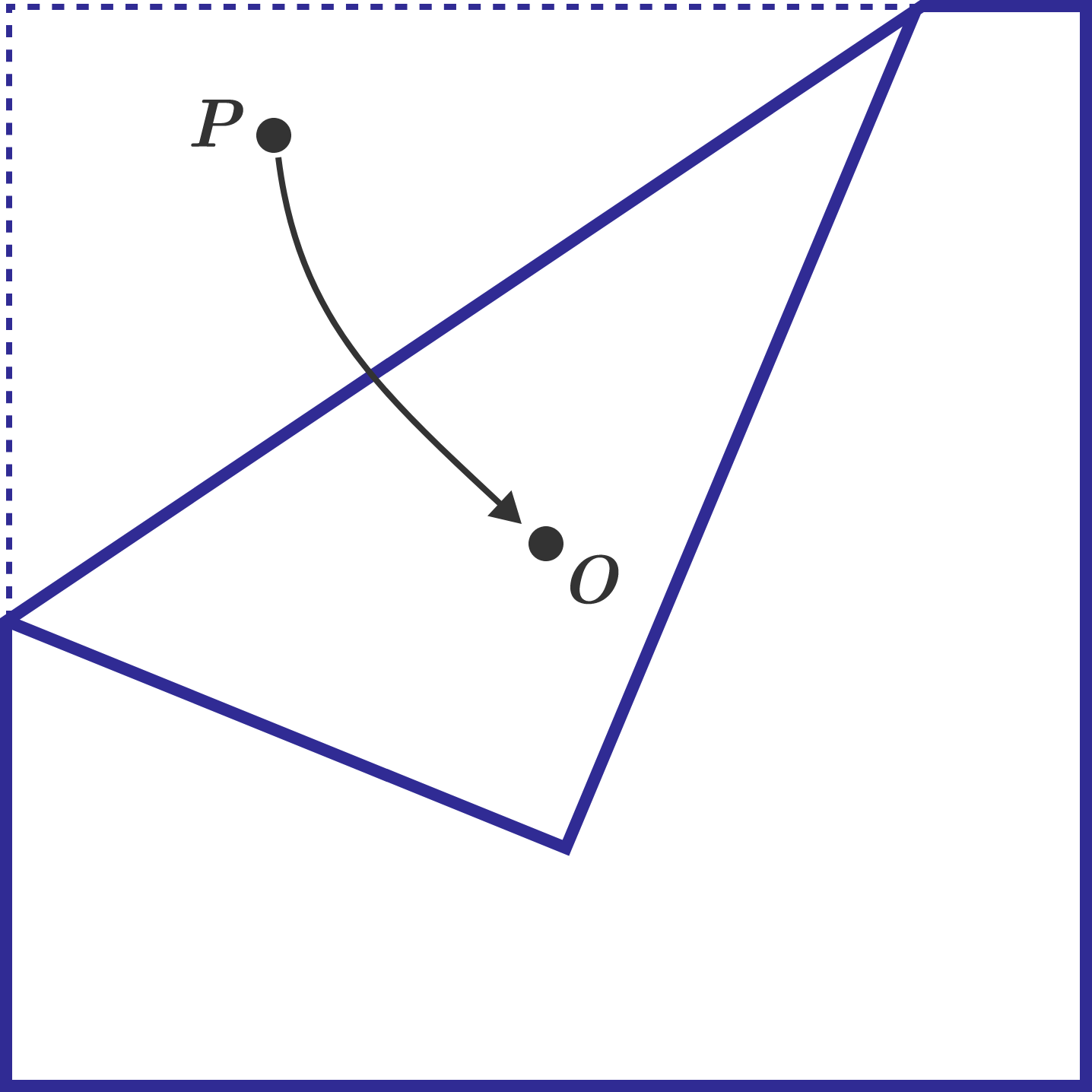# Origami ProbabilityA square sheet of paper has its center marked with point $O$. A random point is uniformly chosen on the square paper, and this point is labeled $P$. The paper is then folded so that point $P$ coincides with point $O$. Let the probability that a pentagon is formed after the fold be $N$. Find the value of $\lfloor 1000N \rfloor$.

×